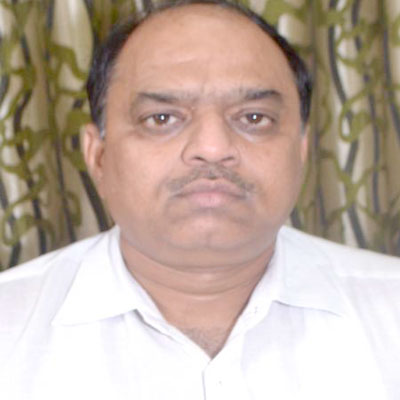# Dr. Sanjay Kumar Singh

Professor
Banaras Hindu University, India

Highest Degree
Ph.D. in Statistics from Banaras Hindu University, Varanasi, Uttar Pradesh, India

# Area of Interest:

Bayesian Inference
100%
Mathematics and Statistics
62%
Statistics
90%
Applied Mathematics
75%
Mathematical Research
55%

Books
0
Chapters
0
Articles
96
Abstracts
2

# Selected Publications

1. Singh, U. and V.K. Sharma, 2014. Estimation on system reliability in generalized lindley stress-strength model. J. Stat. Applic. Probab. Lett., 3: 61-75.
2. Singh, U. and A.S. Yadav, 2014. Bayesian reliability estimation in extension of exponential distribution for progressive type II censored sample with binomial removals using different loss functions. Int. J. Econ. Stat., 13: 19-39.
3. Singh, S.K., U. Singh, M. Kumar and P.K. Vishwakarma, 2014. Classical and bayesian inference for an extension of the exponential distribution under progressive type-II censored data with binomial removals. J. Stat. Applic. Probab. Lett. Int. J., 1: 75-86.
4. Singh, S.K., U. Singh and A.S. Yadav, 2014. Parameter estimation of marshall-olkin exponential distribution under hybrid type-I Censoring scheme. J. Stat. Applic. Probab., 3: 117-127.
5. Singh, S.K., U. Singh and A.S. Yadav, 2014. Bayesian estimation of Marshall-Olkin extended exponential parameters under various approximation techniques. Hacettepe J. Math. Stat., 43: 347-360.
6. Sharma, V.K., S.K. Singh and U. Singh, 2014. A new upside-down bathtub shaped hazard rate model for survival data analysis. Applied Math. Comput., 239: 242-253.
7. Singh, U., G.P. Singh and A. Tripathi, 2013. Bayesian analysis for epidemiological study of child mortality on the district level of Uttar Pradesh. Elixir. Stat., 62: 17901-17907.
8. Singh, U., G.P. Singh and A. Tripathi, 2013. Assessment of effect of socio-economic variables on child mortality through mathematical modeling. JP J. Biostat., 9: 27-38.
9. Singh, U. and V.K. Sharma, 2013. Expected total test time and bayes estimation for the generalized lindley distribution under progressive censoring where removals follow the beta-binomial probability law. Applied Math. Comput., 222: 402-419.
10. Singh, U. and V.K. Sharma, 2013. Bayesian prediction of future observations from inverse weibull distribution based on type-II hybrid censored sample. Int. J. Adv. Stat. Probab., 1: 32-43.
11. Singh, U. and V.K. Sharma, 2013. Bayesian analysis for type-II hybrid censored sample from inverse weibull distribution. Int. J. Syst. Assur. Eng. Manage., 4: 241-248.
12. Singh, U. and M. Kumar, 2013. Estimation of parameters of exponentiated pareto model for progressive type-II censored data with binomial removals using Markov Chain Monte Carlo method. Int. J. Math. Comput., 21: 88-102.
13. Singh, U. and M. Kumar, 2013. Estimation for the parameter of poisson-exponential distribution under Bayesian. J. Data Sci., 12: 157-173.
14. Singh, U. and M. Kumar, 2013. Bayesian inference for exponential distribution based on progressive type- II censored data with random scheme. Elixir online J., 58: 14874-14881.
15. Singh, U. and D. Kumar, 2013. Bayesian estimation of the parameters of generalized inverted exponential distribution. Int. J. Econ. Stat., 1: 32-43.
16. Singh, S.K., U. Singh and D. Kumar, 2013. Bayesian estimation of parameters of inverse weibull distribution. J. Applied Stat., 40: 1597-1607.
17. Singh, U. and D. Kumar, 2011. Estimation of parameters and reliability function of exponentiated exponential distribution: Bayesian approach under general entropy loss function. Pak. J. Stat. Oper. Res. 7: 199-206.
18. Singh, U. and D. Kumar, 2011. Bayesian estimation of the exponentiated gamma parameter and reliability function under asymmetric loss function. Revstat-Stat. J., 9: 247-260.
19. Singh, S.K., U. Singh and G.P. Singh, 2011. Model selection and bayes estimates of the parameter for distribution of waiting time to first birth. Int. J. Curr. Res., 3: 091-095.
20. Singh, G., B.P. Singh, U. Singh and R.D. Singh, 2011. Shrinkage estimator and estimators for shape parameter of classical pareto distribution. J. Scient. Res., 55: 181-207.
21. Singh, R., U. Singh and R.D. Singh, 2009. Bayes estimator of weibull parameters using Lindley's approximation under general entropy loss function. J. Scient. Res., 53: 127-145.
22. Singh, R., U. Singh and G.P. Singh, 2009. Bayes estimators of generalized exponential parameters under general entropy loss function using lindley's approximation. Stat. Trans., 10: 109-127.
23. Singh, S.K., P.K. Singh, S.K Upadhyay and R.D. Singh, 2008. Bayes estimator of weibull parameters under general entropy loss function. J. Sci. Res., 52: 249-262.
24. Singh, R., S.K. Singh, U. Singh and G.P. Singh, 2008. Bayes estimator of generalized exponential parameters under LINEX loss function using lindley's approximation. Data Sci. J., 7: 65-75.
25. Singh, P.K., S.K. Singh and U. Singh, 2008. Bayes estimator of inverse gaussian parameters under general entropy loss function using lindley's approximation. Commun. Stat. Simulation Comput., 37: 1750-1762.